## Expectation Values

Operators allow us to compute the expectation value of some physics quantity given the wavefunction. If a particle is in the state, the normal way to compute the expectation value of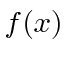isWe can move thebetween just beforeanticipating the use of linear operators.If the variable we wish to compute the expectation value of (like) is not a simple function of, let its operator act on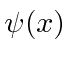. The expectation value ofin the stateis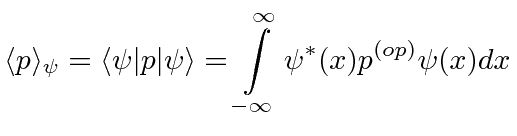The Dirac Bra-ket notation shown above is a convenient way to represent the expectation value of a variable given some state.

* Example: A particle is in the state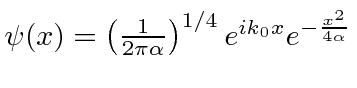. What is the expectation value of?*

For any physical quantity, the expectation value ofin an arbitrary stateis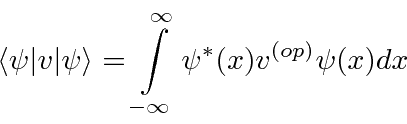The expectation values of physical quantities should be real.

Gasiorowicz Chapter 3

Griffiths Chapter 1

Cohen-Tannoudji et al. Chapter

Jim Branson 2013-04-22